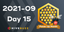On Why the Twin Prime Conjecture is Important

in STEMGeeks4 months ago

HIVE recently attracted a professional mathematician with the handle @petreius who published a paper on the distribution of Twin Primes.

So, I decided to take a short break on philosophy to pen a post on StemGeeks.net explaining why I think the Twin Prime Conjecture is an important piece of mathematics.

The Twin Prime Conjecture is notable as it addresses the difference between arbitrarily large and infinite sets.

A prime number is a positive Integer which is only divisible by itself and the identity element 1. The set starts {2, 3, 5, 7, 11, 13, 17, 19, 23 ...}

Euclid is credited with a proof that there is an infinite number of primes. Lets say we had an arbitrarily large set with the first n primes. Multiplying them together will produce a number that we could denote by P.

This set could have millions upon millions of primes. P could be an incomprehensibly large number. However, we can deduce that the numbers P + 1 and the numbers P - 1 could not possibly be divisible by the first n primes because the number P is a multiple of the first n primes.

Euclid's proof that there is a infinite number of primes is one of the most important and fascinating proofs within number theory.

Anyway, P + 1 and P - 1 happen to be two digits apart. This pair is a potential Twin Prime.

While P is not divisible by the first n primes. It is possible for P + 1 or P - 1 to be divisible by a prime number greater than n. For that matter these numbers are quite often composites.

The twin prime conjecture stipulates that there is an infinite number of twin primes. Euclid's proof produces an infinite set of potential twin primes. But is not quite sufficient to accomplish the task.

The Twin Prime Conjecture and Calculus

You may recall from Calculus that many proofs involve arbitrarily large sets of numbers. In many cases, like Euclid's conjecture, it is sufficient to prove the statement true for an arbitrarily large set of numbers. In a few cases like the Twin Prime conjecture it is not.

I believe it is possible to contrive a proof that is true for an arbitrarily large set of numbers, but is not true for an infinite set.

I recall contriving such an example a long time ago, but I forgot the example. Perhaps it is possible to do something with Cantor's diagonal method. But that would be a different post.

Many people see the Twin Prime Conjecture as some sort of esoteric piece of mathematics trivia. I am fascinated by the conjecture because it touches on some of the most important concepts in mathematics.

I couldn't figure out how to make a picture that visualizes the twin prime conjecture; So I snagged a picture from Creative Commons that show two twins in their prime discussing mathematics.

They must be discussing mathematics. One is holding something that looks a bit like a calculator and has an extended finger to make a point.Posted with STEMGeeks

Sort:

Congratulations @yintercept! You have completed the following achievement on the Hive blockchain and have been rewarded with new badge(s) :You received more than 8000 upvotes.Your next target is to reach 9000 upvotes.Hive Power Up Month - Feedback from Day 15